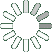Puzzle Command
Daily Puzzle
Number Logic
November 27, 2014Find a 6 digit number where:

• The 1st digit is the sum of the 3rd and 4th digit.
• The 2nd digit is the number of 0s in the number.
• The 3rd digit is 6.
• The 4th digit is the number of 2s in the number.
• The 5th digit is 0.
• The 6th digit is the number of 3s in the number.
Puzzle Notes
The 1st digit is the sum of the 3rd and 4th digit.
The 2nd digit is the number of 0s in the number.
The 3rd digit is 6.
The 4th digit is the number of 2s in the number.
The 5th digit is 0.
The 6th digit is the number of 3s in the number.
Enter in your solution below and click submit.